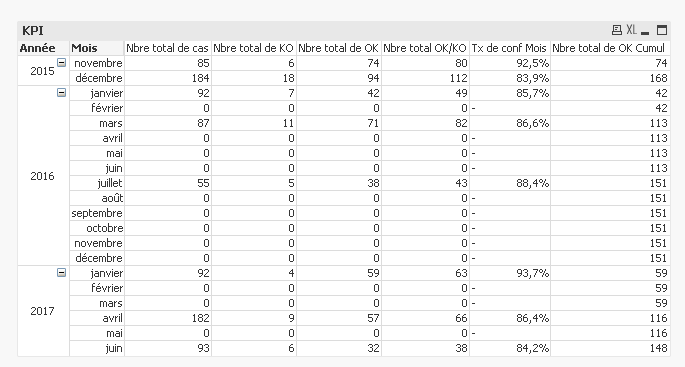# QlikView App Dev

Discussion Board for collaboration related to QlikView App Development.

Announcements
On May 18th at 10AM EDT we will answer your QlikView questions live. REGISTER
cancel
Showing results for
Did you mean:Not applicable

## Cumulative sum in a two-dimensional pivot table

Hello,

Here is my pivot table :There are two dimensions year (Année) and month (mois)  and I'm currently trying to calculate a cumulative sum for the column "Nbre total de OK".

The cumulative sum is stored in the column "Nbre total de OK Cumul", as you can see I already tried something with this expression : rangesum(above( sum([Nbre total de OK]), 0, rowno())) .

The problem is that it restarts the sum for each year, any idea how to just have the cumulative sum without restarting ?

1 Solution

Accepted SolutionsNot applicable
Author

Here is the correct expression : RangeSum(Above(total sum([Nbre total de OK]), 0, RowNo(TOTAL)))